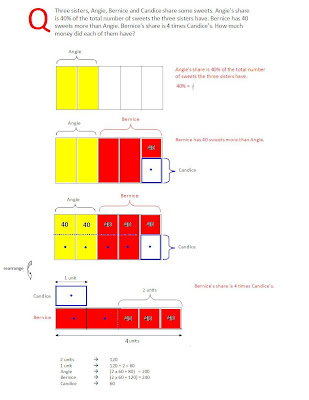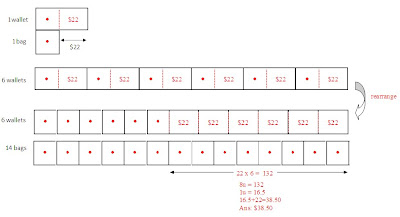## Tuesday, March 16

### The Maths Challenge to Parent - UTM book Q5 Pg 20

Hi Sunny

My daughter was trying out the Q5 on page 20 of your UTM book. I have download the answers and explanation, but I don't seem to understand what the "40" in the boxes that you have placed in the diagram. How did you derive the "40"?

Question: Three sisters, Angie, Bernice and Candice share some sweets. Angie's share is 40% of the total number of sweets the three sisters have. Bernice has 40 sweets more than Angie. Bernice's share is 4 times Candice’s. How much money did each of them have?

Thanks

Vijay
From the Desk of Sunny Tan........
The explanation in the book use conventional model. I will illustrate visualizing the model in another perspective - stack model:## Tuesday, March 9

### Conventional Model VS Stack Model

In my seminars, the following are the most common questions raised by many parents;

a) What are the differences between conventional and stack Model approach?
b) Which model is the more “superior” approach?
c) Will it be confusing for the child to “switch” from conventional to stack model?

Many parents have the misconception that stack Model is completely new approach, that is different from the conventional model. I would like to emphasis that Stack model and conventional Model uses the same fundamental concepts of units to solve problem. Basically, they are the same. The only MAIN difference is the orientation of drawing the model.

Stack model utilizes visual processing technique – enable the child to minimize the movement (rearrange) the models and to combine all the steps required in the conventional model into a single step by using a single model. Then objective is to enable the child to “see” the problems laterally!

I will use the below example to illustrate my point:

The total cost of 14 bags is equal to the total cost of 6 wallets. Each wallet costs \$22 more than each bag. Find the cost of each wallet.

CONVENTIONAL MODEL:STACK MODEL: